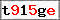This website uses cookies to improve user experience. By using our website you consent to all cookies in accordance with our Cookie Policy. X

### 3D engine for beginners III [advanced]

In previous parts of this guide dedicated to creating a very bare-bone 3D engine I've mentioned how to display the illusion of perspective and how to control camera movements. This time we will handle rotation. It will be the final chapter of this guide since any further immersion in the topic will require knowledge of more advanced issues, which are better to handle with a "proper" engine (like for example Alternativa3D or Away3D). Not to mention the newest Flash version with number 11 was extended with 3D acceleration, which basically means ActionScript can now be used to create a proper 3D game in likes of Crysis or Gears of War (especially since Adobe presented Unreal Engine 3 working in Flash).
But that is a different story, for now let's focus on that rotation.

1. However before we do anything create two new constants and one variable:

```const SPEED:Number = 20;
const ROTATION:Number = Math.PI/16;
var a:Number = 0;
```
SPEED will be the speed of camera movement, while ROTATION will be the speed of rotation. The a variable will be used to store current rotation angle.

2. Do you remember which part of the code was responsible for transformation from 3D into 2D? We will modify it to include turning of the camera:

```var nx:Number;
var nz:Number;
var rx:Number;
var rz:Number;
var bx2:Array = new Array(bx.length);
var by2:Array = new Array(by.length);
var i:Number = 0;
while(i<bz.length) {
nx = cx-bx[i];
nz = cz-bz[i];
rx = cx+( nx*Math.cos(-a) )-( nz*Math.sin(-a) );
rz = cz+( nx*Math.sin(-a) )+( nz*Math.cos(-a) );
bx2[i] = vw+( ((cx-rx) / (cz-rz) )*vw);
by2[i] = vh+( ((cy-by[i]) / (cz-rz) )*vh);
++i;
}
```
Not much of a change, I've added additional variables for better clarity and the rotation formula (along the Y axis, which is exactly the same as in any other FPS game). You will probably find nothing shocking here if math is no stranger to you. Long story short for each point we calculate its translation (in case of x: nx = cx-bx[i];), which is rotated (( nx*Math.cos(-a) )-( nz*Math.sin(-a) );) and put back into position ( rx = cx+...). Newly created point is then transformed from 3D into 2D using the method we are already familiar with.

3. Now the only thing left to do is to modify the camera movement to include an option for turning around. We will accomplish this by changing how key presses are translated into actions by the switch(key) command. It is also a good opportunity to optimise the code - replace it with this:

```switch(key&0x0000ff) {
case 0x00000f:
cz += Math.cos(-a)*SPEED;
cx += Math.sin(-a)*SPEED;
break;
case 0x0000f0:
cz -= Math.cos(-a)*SPEED;
cx -= Math.sin(-a)*SPEED;
break;
}
switch(key&0x00ff00) {
case 0x000f00:
a += ROTATION;
break;
case 0x00f000:
a -= ROTATION;
break;
}
```
In the previous version switch was used to check every possible combination within the key variable, while now switch extracts only a part of key - first one gets up and down keys (0x000f0f&0x0000ff will result in 0x00000f which means the information about the left key, 0x000f00, is omitted), while the second one gets left and right.
Okay, so now we handle the key presses better, but what is actually happening here? Let's start from the end: left and right keys are used to turn (change the angle), while up and down are used for forward and backward movement. Of course since now we have to deal with rotation, the definition of "forward" constantly changes and that is why we need to use cos and sin functions which in this case calculate the translation vector based on the angle.

4. Time to test this out. Final result should look more or less like this:
(Movement with arrow keys)

And the whole class is available here: box3d_rot.zip

Name:
Comment:
Confirm the image code: